# Excercise 3.3 Understanding Quadrilaterals- NCERT Solutions Class 8

## Chapter 3 Ex.3.3 Question 1

Given a parallelogram $$ABCD$$. Complete each statement along with the definition or property used.

(i)$$\, AD\, = \,\_\_\_\_\_\_\_\_\_$$

(ii)$$\, \angle DCB\, = \,\_\_\_\_\_\_$$

(iii)$$\,OC\, = \,\_\_\_\_\_\_\_\_$$

(iv) $$\,m\,\angle DAB\,\!\!+\!\!\,m\,\angle CDA\,\!\!=\_\_\_\_\_\_\_$$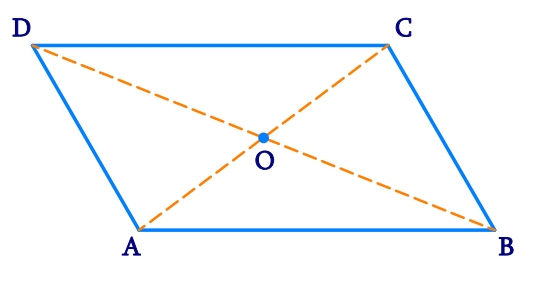### Solution

What is Known?

$$ABCD$$ is a parallelogram.

What is Unknown?

\begin{align}&{\rm{AD}},\angle DCB,\,{\rm{OC}},\,\,\\&{\rm{m}}\angle DAB +\!{\rm{m}}\angle CDA\end{align}

Reasoning:

We can use the properties of parallelogram to determine the solution.

Steps:

i) The opposite sides of a parallelogram are of equal length.

$${\rm{AD }} = {\rm{ BC}}$$

(ii) In a parallelogram, opposite angles are equal in measure.

$$\angle {\rm{DCB }} = {\rm{ }}\angle {\rm{DAB}}$$

(iii) In a parallelogram, diagonals bisect each other. Hence,

$${\rm{OC }} = {\rm{ OA}}$$

(iv)In a parallelogram, adjacent angles are supplementary to each other. Hence,

$$m\angle {\rm{DAB }} + {\rm{ m}}\angle {\rm{CDA }} = {\rm{18}}0^\circ$$

## Chapter 3 Ex.3.3 Question 2

Consider the following parallelograms. Find the values of the unknowns $$x$$, $$y$$, $$z$$.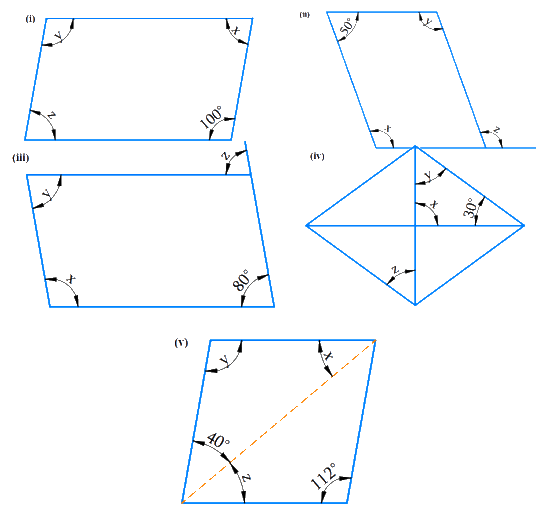### Solution

i) What is Known?

$$ABCD$$ is a parallelogram.

What is Unknown?

Values of $$x$$, $$y$$, $$z$$.

Reasoning:

In a parallelogram, opposite angles are equal and adjacent angles are supplementary. Using this property, we can calculate the measure of unknown angles.

Steps: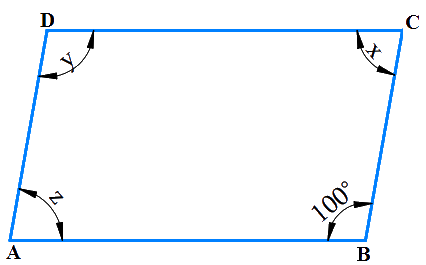Since $$D$$ is opposite to $$B$$.

So, $${{y }} = {\rm{ 1}}{00^{\rm{o}}}$$ [Since opposite angles of a parallelogram are equal]

$$\angle C + \angle {\rm{B}} = {\rm{18}}0^\circ$$(The adjacent angles in a parallelogram are supplementary)

$${{x }} + {\rm{ 1}}00^\circ {\rm{ }} = {\rm{ 18}}0^\circ {\rm{ }}$$ (The adjacent angles in a parallelogram are supplementary)

Therefore,

\begin{align} x & ={{180}^{{}^\circ }}-{{100}^{{}^\circ }} \\ {} & ={{80}^{{}^\circ }} \\\end{align}

$${{x}} = {{ z}} = {\rm{8}}0^\circ {\rm{ }}$$ [Since opposite angles of a parallelogram are equal]

ii) What is Known?

Given figure is a parallelogram.

What is Unknown?

values of $$x$$, $$y$$, $$z$$.

Reasoning:

In a parallelogram, opposite angles are equal and adjacent angles are supplementary. Using this property, we can calculate the measure of the unknown angles.

Steps: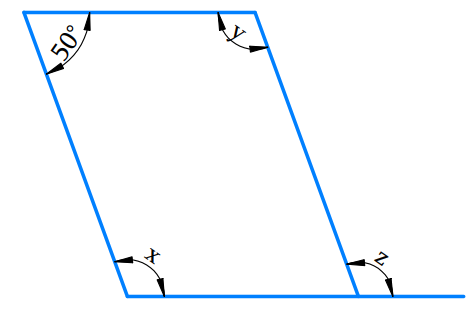\begin{align}{{\text{x}} + {\rm{5}}{0^\circ }}&{ = {\rm{18}}{0^\circ }\left( \begin{array}{l}{\text{The adjacent angles}}\\{\text{ in a parallelogram }}\\{\text{are supplementary}}\end{array} \right)}\\ {\rm{x}}&{ = {\rm{18}}{0^\circ } - {\rm{5}}{0^\circ }}\\&{ = {\rm{13}}{0^\circ }}\end{align}

\begin{align} & \text{x }\!=\!\text{y}\!=\!\text{13}0^\circ \left( \begin{array}{l}{\text{Since opposite angles}}\\{\text{ of a parallelogram }}\\{\text{are equal}}\end{array} \right) \\& \text{x}=\text{z}=\text{13}0^\circ \text{(Corresponding angles)} \\\end{align}

iii) What is Known?

Given figure is a parallelogram.

What is Unknown?

values of $$x$$, $$y$$, $$z$$.

Reasoning:

In a parallelogram, opposite angles are equal and adjacent angles are supplementary. Using this property, we can calculate the measure of the unknown angles

Steps: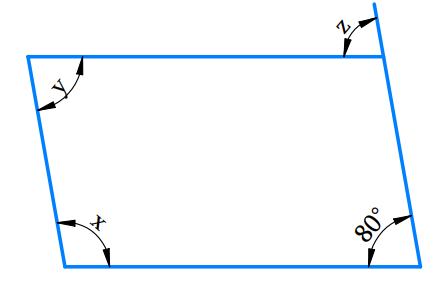\begin{align}z&={{80}^{\text{o}}}\text{ (Corresponding angles) } \\y&={{80}^{\text{o}}}\left( \begin{array}{l}{\text{since opposite angles}}\\{\text{ of a parallelogram }}\\{\text{are equal}}\end{array} \right) \\x+y&={{180}^{\text{o}}}\left( \begin{array}{l}{\text{Adjacent angles }}\\{\text{are supplementary}}\end{array} \right)\\x+{{80}^{\text{o}}}&={{180}^{\text{o}}} \\x&={{180}^{\text{o}}}-{{80}^{\text{o}}} \\x&={{100}^{{}^\circ }} \\\end{align}

$\text{Therefore x}={{100}^{{}^\circ }},\,\,\,\text{y}={{80}^{{}^\circ }},\,\,\,\text{z}={{80}^{{}^\circ }}$

iv) What is the known?

Given figure is a parallelogram.

What is unknown?

Values of $$x$$, $$y$$, $$z$$.

Reasoning:

In a parallelogram, opposite angles are equal and adjacent angles are supplementary. Using this property, we can calculate the measure of the unknown angles.

Steps: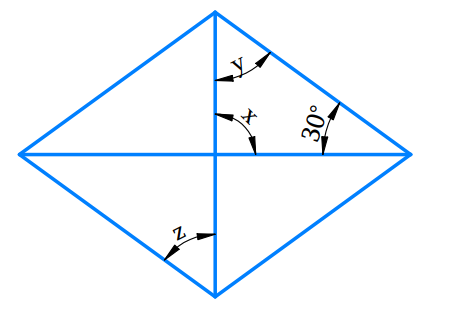\begin{align}\text{x}+\text{y}+{{30}^{\text{o}}}&={{180}^{\text{o}}}\left( \begin{array}{l}{\text{Angle sum}}\\{\text{ property of }}\\{\text{triangles}}\end{array} \right)\\ \text{x}&\!\!=\!\!{{90}^{\text{o}}}\left( \begin{array}{l}{\text{Vertically }}\\{\text{opposite angles}}\end{array} \right)\\ {{90}^{{}^\circ }}+\text{y}+{{30}^{\text{o}}}&={{180}^{\text{o}}} \\ \text{y}+120&={{180}^{\text{o}}} \\ \text{y}&={{180}^{\text{o}}} \\ \text{z}&={{60}^{\text{o}}}\end{align}

Therefore

$\text{z}=\text{y}={{60}^{\text{o}}}\left( \begin{array}{l}{\text{Alternate interior }}\\{\text{angles are equal}}\end{array} \right)$

v) What is Known?

Given figure is a parallelogram.

What is Unknown?

Values of $$x$$, $$y$$, $$z$$.

Reasoning:

In parallelogram opposite angles are equal and Adjacent angles are supplementary.

Using this property, we can calculate the unknown angles.

Steps: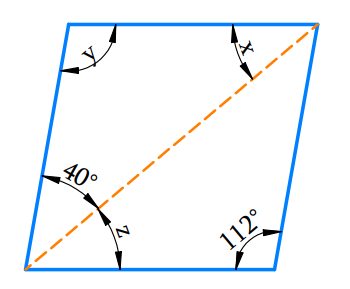$$y=112^\circ$$(Since opposite angles of a parallelogram are equal)

\begin{align}x+y+40^\circ&=180^\circ\\\text{(Angle sum }&\text{property of triangles)} \end{align}

\begin{align}{x}+{{112}^{\circ}}+{{40}^{\circ}}&={{180}^{\circ}} \\{x}+{{152}^{\circ}}&={{180}^{\circ}} \\ {x}&={{180}^{\circ}}-{{152}^{\circ}} \\ {x}&={{28}^{\circ}} \\{z}&={x}={{28}^{\circ}}\\\text{ (Alternate}&\text{ interior angles)}\end{align}

Therefore,

\begin{align}x &={{28}^{{}^\circ }},{y}={{112}^{{}^\circ }},{z}={{28}^{{}^\circ }} \\\end{align}

## Chapter 3 Ex.3.3 Question 3

Can a quadrilateral $$ABCD$$ be a parallelogram if

(i) $$∠D +∠B = 180^\circ?$$

(ii) $$AB = DC = 8 \rm \,cm, AD = 4 \rm \,cm$$ and $$BC = 4.4 \rm \,cm?$$

(iii) $$∠A = 70^\circ$$ and  $$∠C = 65^\circ?$$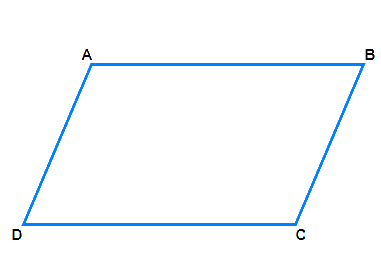### Solution

i) What is Known?

Given figure is a quadrilateral

What is Unknown?

If $$ABCD$$ is a parallelogram when $$\angle {\rm{D }} + \angle {\rm{B }} = {\rm{18}}0^\circ ?$$

Reasoning:

A parallelogram is a quadrilateral whose opposite sides are parallel.

In parallelogram opposite angles are equal and adjacent angles are supplementary.

Using this property, we can calculate the unknown angles.

Steps:

Using the angle sum property of a quadrilateral,

\begin{align}\angle A+\angle B+\angle D+\angle C&={{360}^{\circ}} \\\angle A+\angle C+{{180}^{\circ}}&={{360}^{\circ}} \\\angle A+\angle C&={{360}^{\circ}}-{{180}^{\circ}} \\\ \angle A+\angle C&={{180}^{\circ}}\end{align}

(Opposite angles should also be of same measures.)

For $$\angle {\rm{D}} + \angle {\rm{B}}$$ $$= {\rm{18}}0^\circ$$, is a parallelogram.

If the following conditions is fulfilled, then $$ABCD$$ is a parallelogram.

The sum of the measures of the adjacent angles should be $$180^\circ$$.

Opposite angles should also be of same measure.

ii) What is Known?

Given figure is a quadrilateral.

What is Unknown?

$$ABCD$$ be a parallelogram if $$AB = DC = 8 \rm \,cm, AD = 4 \rm \,cm$$ and $$BC = 4.4 \rm \,cm$$

Reasoning:

A parallelogram is a quadrilateral whose opposite sides are parallel.

Steps:

Property of parallelogram: The opposite sides of a parallelogram are of equal length. Opposite sides $$AD$$ and $$BC$$ are of different lengths. So, it’s not parallelogram.

iii) What is Known?

Given figure is a quadrilateral.

What is Unknown?

$$ABCD$$ be a parallelogram if $$\angle {\rm{A }} = {\rm{ 7}}0^\circ {\rm{ }}$$ and $$\angle {\rm{C }} = {\rm{ 65}}^\circ ?$$

Reasoning:

A parallelogram is a quadrilateral whose opposite sides and angles are equal.

Steps:

Property: In a parallelogram opposite angles are equal.

So, $$\angle {\rm{A }} = {\rm{ 7}}0^\circ {\rm{ }}$$and $$\angle {\rm{C }} = {\rm{ 65}}^\circ {\rm{ }}$$are not equal.

So $$ABCD$$ is not parallelogram.

## Chapter 3 Ex.3.3 Question 4

Draw a rough figure of a quadrilateral that is not a parallelogram but has exactly two opposite angles of equal measure.

### Solution

What is Known?

Draw a figure of quadrilateral having two opposite angles of equal measure.

What is Unknown?

$$ABCD$$ is quadrilateral whose opposite angles are equal.

Reasoning:

The opposite angles of a parallelogram are equal.

Steps: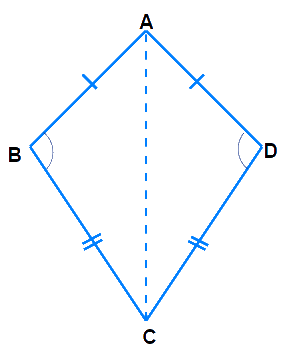In a kite, the angle between unequal sides are equal.

Draw line from $$A$$ to $$C$$ and we will get two triangles with common base $$AC$$.

In $$∆ABC$$ and $$∆ADC$$ we have,

$${\rm{AB}} = {\rm{ AD }},{\rm{ BC}} = {\rm{CD}}$$ ; $$AC$$ is common to both

$$\Delta {\rm{ABC }}\!\!\cong\!\!\Delta {\rm{ADC }}\text{[congruent triangles]}$$

Hence corresponding parts of congruent triangles are equal.

Therefore$$\angle {\rm{B}} = \angle {\rm{D}}$$

However, the quadrilateral $$ABCD$$ is not a parallelogram as the measures of the remaining pair of opposite angles, $$\angle {\rm{A}}$$ and $$\angle {\rm{C}}$$, are not equal. Since they form angle between equal sides.

## Chapter 3 Ex.3.3 Question 5

The measures of two adjacent angles of a parallelogram are in the ratio $${\rm{3}}:{\rm{2}}$$. Find the measure of each of the angles of the parallelogram.

### Solution

What is Known?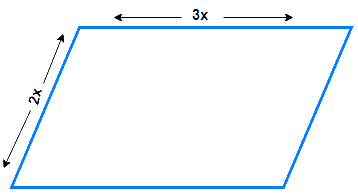Given figure is a parallelogram and two adjacent angles are having ratio of $${\rm{3}}:{\rm{2}}$$ quadrilateral.

What is Unknown?

Measure of Each angles of parallelogram.

Reasoning:

A parallelogram is a quadrilateral whose opposite angles are equal.

Steps:

We know that the sum of the measures of adjacent angles is $$180º$$ for a parallelogram.

\begin{align}\angle A + \angle B &= {180^{\rm{o}}}\\3x + 2x &= {180^{\rm{o}}}\\5x &= {180^{\rm{o}}}\\x &= \frac{{{{180}^{\rm{o}}}}}{5}\\x &= \,{36^{\rm{o}}}\end{align}

\begin{align} \angle A&=\angle C=3x \\ & ={{108}^{\circ}}\text{ }(\text{ Opposite angles }) \\ \angle B&=\angle D=2x \\ & ={{72}^{\circ}}\text{ (Opposite angles) } \end{align}

Thus, the measures of the angles of the parallelogram are $$108^\circ, 72^\circ, 108^\circ,$$ and $$72^\circ$$.

## Chapter 3 Ex.3.3 Question 6

Two adjacent angles of a parallelogram have equal measure. Find the measure of each of the angles of the parallelogram.

### Solution

What is Known?

Two adjacent angles of a parallelogram have equal measure.

What is Unknown?

Measure of each of the angles of the parallelogram.

Reasoning:

In parallelogram opposite angles are equal and adjacent angles are supplementary.

Using this property, we can calculate the unknown angles.

Steps: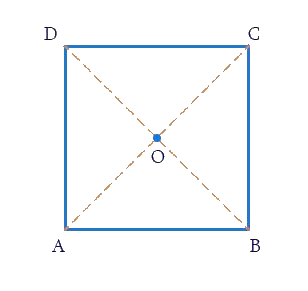In parallelogram $$ABCD$$,

$$\angle {\rm{A }}$$ and $$\angle {\rm{D}}$$ are supplementary since $$DC$$ is parallel to $$AB$$ and with transversal $$DA$$,

making $$\angle {\rm{A}}$$ and $$\angle D$$ interior opposite.

$$\angle {\rm{A }}$$ and $$\angle {\rm{B }}$$ are also supplementary since $$AD$$ is parallel to $$BC$$ and with transversal $$BA$$, making $$\angle {\rm{A}}$$ and $$\angle {\rm{B}}$$ interior opposite.

Sum of adjacent angles $$= {\rm{18}}0^\circ$$

Let each adjacent angle be $$x$$

Since the adjacent angles in a parallelogram are supplementary.

\begin{align}x + x &= 180^\circ \\2x &= 180^\circ \\x &= \frac{{{\rm{18}}0^\circ }}{2}\end{align}

Hence, each adjacent angle is $$90$$.

\begin{align}\angle \text{A }&\!\!=\!\!\angle \text{B}\!\!=\!\!\text{ 9}0{}^\text{o}\text{ }\left( \text{adjacent angles} \right) \\ \angle \text{C }&\!\!=\!\!\angle \text{A}\!\!=\!\!\text{ 9}0{}^\text{o}\text{ }\left( \text{Opposite angles} \right) \\ \angle \text{D}&\!\!=\!\!\angle \text{B}\!\!=\!\!\text{9}0{}^\text{o}\left( \text{Opposite angles} \right) \end{align}

Thus, each angle of the parallelogram measures $$90^\circ$$.

## Chapter 3 Ex.3.3 Question 7

The adjacent figure $$HOPE$$ is a parallelogram. Find the angle measures $$x, y$$ and $$z.$$ State the properties you use to find them.

### Solution

What is Known?

Given figure is a parallelogram.

What is Unknown?

Values of $$x, y, z.$$

Reasoning:

In a parallelogram, opposite angles are equal and adjacent angles are supplementary. Using this property, we can calculate the unknown angles.

Steps: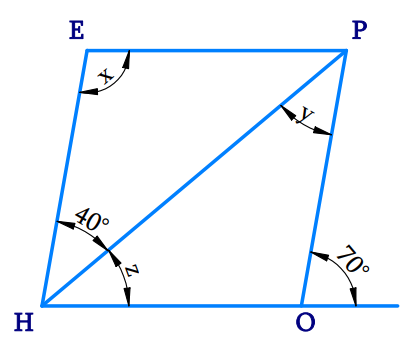Here,

\begin{align}\angle &{\rm{HOP }} + {\rm{ 7}}0^\circ = {\rm{ 18}}0^\circ {\rm{ }}\,\,\\&\left[ {{\text{Angles of linear pair}}} \right]\\\angle &{\rm{HOP}} = {\rm{18}}0^\circ - {\rm{7}}0^\circ \\\angle &{\rm{HOP}} = {\rm{11}}0^\circ \\\angle &{\rm{O }} = \angle E\left( {{\text{opposite angles are equal}}} \right)\\\therefore &{\rm{x}} = {\rm{11}}0^\circ {\rm{ }}\end{align}

\begin{align}\text{y}&={{40}^{\text{o}}}\\\text{ (Alternate }&\text{interior angles are equal) } \\\text{z}+{{40}^{\circ}}&={{70}^{\circ}}\\&\text{ (Corresponding angles) } \\ \text{z}&={{70}^{\circ}}-{{40}^{\circ}} \\ \text{z}&={{30}^{\circ}} \\ \end{align}

$\therefore {\text{ x }} = {\rm{ 11}}0^\circ ,{\text{ y }} = {\text{ 4}}0^\circ ,{\rm{ z }} = {\text{ 3}}0^\circ$

## Chapter 3 Ex.3.3 Question 8

The following figures $$GUNS$$ and $$RUNS$$ are parallelograms. Find $$x$$ and $$y$$. (Lengths are in $$\rm cm$$)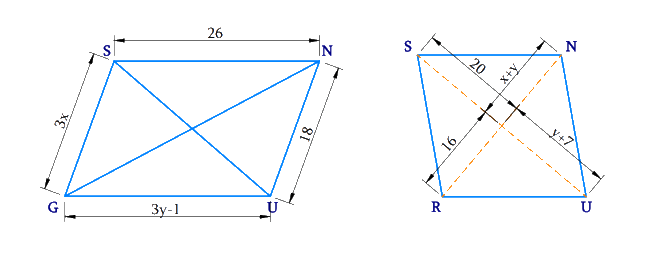### Solution

i) What is Known?

Given figure is a parallelogram.

What is Unknown?

Values of $$x$$, $$y$$

Reasoning:

The diagonals of a parallelogram bisect each other, in a parallelogram, the opposite sides have same length.

Steps: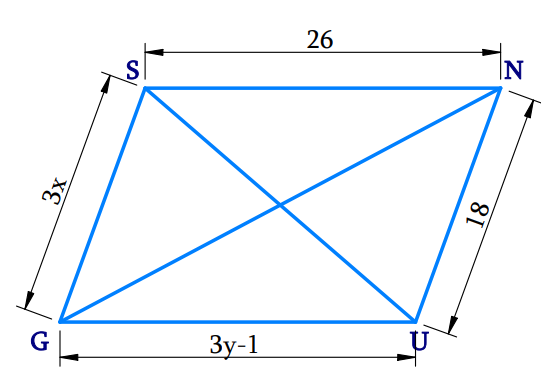In a parallelogram, the opposite sides have same length.

\begin{align}{\rm{SG}}\,\rm&= \,{\rm{NU}}\\3x &= 18\\x &= \,\frac{{18}}{3}\\x &= 6\end{align}

And,

\begin{align}\text{SN}&=\text{GU} \\ 26&=3y-1 \\ 3y&=26+1 \\y&=\frac{27}{3} \\y&=9 \\\end{align}

Hence, the measures of $$x$$ and $$y$$ are $$6 \rm\, cm$$ and $$9 \rm \,cm$$ respectively.

(ii) What is Known?

Given figure is a parallelogram.

What is Unknown?

Values of $$x, y$$

Reasoning:

The diagonals of a parallelogram bisect each other. In a parallelogram, the opposite sides have same length.

Steps: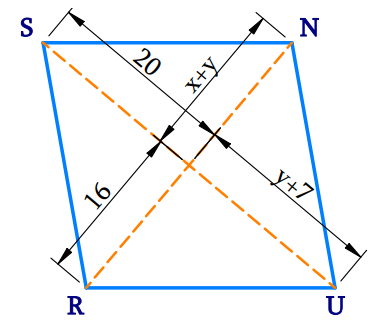Property: The diagonals of a parallelogram bisect each other.

\begin{align}y{\rm{ }} + {\rm{ }}7{\rm{ }} &= {\rm{ }}20\\y &= 20 - 7\\y{\rm{ }} &= {\rm{ }}13\\ x{\rm{ }} + {\rm{ }}y{\rm{ }} &= {\rm{ }}16\\x{\rm{ }} + {\rm{ }}13{\rm{ }} &= {\rm{ }}16\\x{\rm{ }} &= {\rm{ }}3\end{align}

Hence, the measures of $$x$$ and $$y$$ are $$3 \rm\,cm$$ and $$13 \rm\,cm$$ respectively.

## Chapter 3 Ex.3.3 Question 9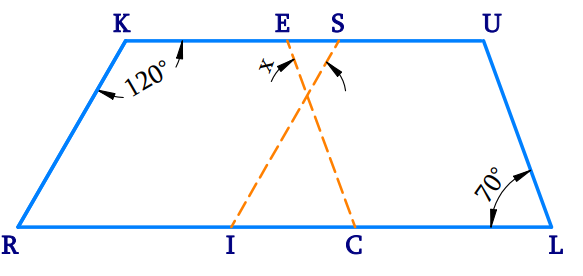In the above figure both $$RISK$$ and $$CLUE$$ are parallelograms. Find the value of $$x$$.

### Solution

What is Known?

In the given figure $$RISK$$and $$CLUE$$ are parallelograms.

What is Unknown?

Values of $$x$$

Reasoning:

The diagonals of a parallelogram bisect each other. Also, in a parallelogram, opposite angles are equal and adjacent angles are supplementary. Using this property, we can calculate the unknown angles.

Steps:In parallelogram $$RISK$$

$${\rm{RKS }} + \angle {\rm{ISK }} = {\rm{ 18}}0^\circ$$  (Adjacent angles of a parallelogram are supplementary)

\begin{align}{\rm{12}}0^\circ + \,\angle {\rm{ISK }} &= {\rm{18}}0^\circ \\\angle {\rm{ISK }} &= {\rm{ 6}}0^\circ\end{align}

\begin{align}{\angle {\text{I}}}&{ = \angle {\rm{K}}}\,{\left( \begin{array}{l}{\text{In parallelogram opposite }}\\{\text{angles are equal}}\end{array} \right)}\\&{ = {\rm{12}}{0^\circ }}&\end{align}

In parallelogram $$CLUE$$

\begin{align}{\angle {\rm{L}}}&{ = \angle {\rm{E}}}\,{\left( \begin{array}{l}{\text{In parallelogram opposite}}\\{\text{ angles are equal}}\end{array} \right)}\\&{ = {\rm{7}}{0^\circ }}&\end{align}

The sum of the measures of all the interior angles of a triangle is $$180^\circ.$$

\begin{align}x+{{60}^{\circ}}+{{70}^{\circ}}&={{180}^{\circ}} \\x+130&={{180}^{\circ}} \\x&={{180}^{\circ}}-{{130}^{\circ}} \\x&={{50}^{\circ}} \\\end{align}

## Chapter 3 Ex.3.3 Question 10

Explain how this figure is a trapezium. Which of its two sides are parallel?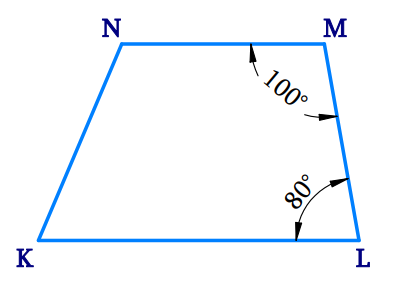### Solution

What is Known?

Given figure is a Quadrilateral.

What is Unknown?

To identify the two parallel sides of the figure and to prove that it is a trapezium.

Reasoning:

Trapezium is a quadrilateral having one pair of parallel sides.

Steps:

In the given figure $$KLMN$$,

$$\angle {\rm{L }}\,+$$ $${\rm{ }}\angle {\rm{M }}$$ $$= {\rm{18}}0^\circ$$ [two pair of adjacent angles (which form pairs of consecutive interior angles) are supplementary]

$= {\rm{ 8}}0^\circ {\rm{ }} + {\rm{1}}00^\circ = {\rm{18}}0^\circ$

Therefore, $$KN$$ is parallel to $$ML$$

Hence, $$KLMN$$ is a trapezium as it has a pair of parallel sides: $$KN$$ and $$ML$$.

## Chapter 3 Ex.3.3 Question 11

Find $${\rm{m}}\,\angle {\rm{C }}$$ in Fig $$3.33$$ if $$AB$$ is parallel to $$DC$$.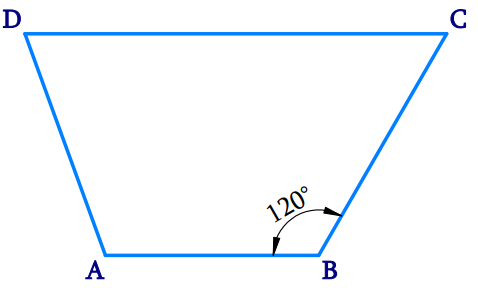### Solution

What is Known?

Given figure is a Quadrilateral with two sides running parallel and one angle is given.

What is Unknown?

Find $${\rm{m }}\angle {\rm{C}}$$

Reasoning:

Trapezium is a quadrilateral with one pair of parallel sides.

Steps:Given figure $$ABCD$$ is a Trapezium, in which $$AB$$ is parallel to $$DC$$.

Here,

\begin{align}\angle {\rm{B}} + \angle {\rm{C}} &= {180^ \circ }\left[ \begin{array}{l}{\text{pair of adjacent }}\\{\text{angles are }}\\{\text{supplementary}}\end{array} \right]\\{\rm{12}}{0^\circ } + \angle {\rm{C}} &= {180^ \circ }\\\angle {\rm{C}} &= {180^ \circ }\\\angle {\rm{C}} &= {180^ \circ } - {120^ \circ }\\\angle {\rm{C }}&={60^ \circ }\\\therefore \,\,m\angle {\rm{C }}&={60^ \circ }\end{align}

## Chapter 3 Ex.3.3 Question 12

Find the measure of $$\angle {\rm{P }}$$ and $$\angle {\rm{S}}$$ if $$SP$$ is parallel to $$RQ$$ in Fig .

(If you find $${\rm{m}}\angle {\rm{R}}$$, is there more than one method to find $${\rm{m}}\angle {\rm{P}}\,?$$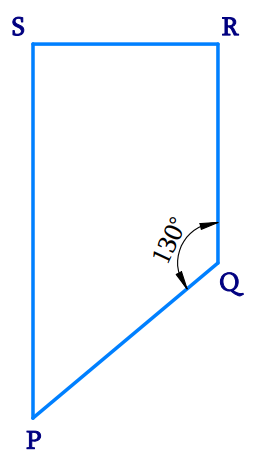### Solution

What is Known?

Given figure is a Quadrilateral.

What is Unknown?

Find $${\rm{m}}\angle P\,{\rm{ and}\, {m}}\angle S$$

Reasoning:

Sum of the measures of all the interior angles of a quadrilateral is $$360^\circ$$.

Steps:Given $$SP$$ is parallel $$RQ$$ and $$SR$$ is the traversal drawn to these lines. Hence,

\begin{align}\angle \text{S}+\angle \text{R}&={{180}^{\text{o}}}\\\angle \text{S}+{{90}^{\circ}}&={{180}^{\circ}} \\\angle \text{S}&={{180}^{\circ}}-{{90}^{\circ}} \\\angle \text{S}&={{90}^{\circ}} \\ \end{align}

Using the angle sum property of a quadrilateral,

\begin{align}\angle \text{S}+\angle \text{P}+\angle \text{Q}+\angle \text{R}&={{360}^{\text{o}}} \\{{90}^{\text{o}}}+\angle \text{P}+{{130}^{\text{o}}}+{{90}^{\text{o}}}&={{360}^{\text{o}}} \\\angle \text{P}+{{310}^{\text{o}}}&={{360}^{\text{o}}} \\\angle \text{P}&\!\!=\!{{360}^{\text{o}}}\!\!-\!\!{{310}^{\text{o}}} \\\angle \text{P}&={{50}^{\text{o}}} \\\end{align}

Instant doubt clearing with Cuemath Advanced Math Program